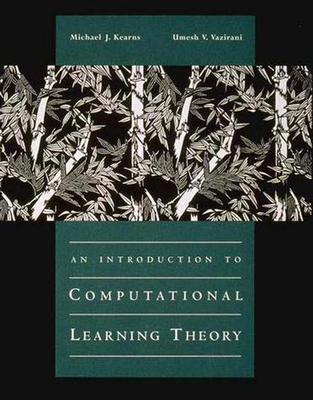### An Introduction to Computational Learning Theory### Huge savings for students

Each student receives a 50% discount off of most books in the HSG Book Store. During class, please ask the instructor about purchase details.
 List Price: \$70.00 Price: \$35.00 You Save: \$35.00
4Emphasizing issues of computational efficiency, Michael Kearns and Umesh Vazirani introduce a number of central topics in computational learning theory for researchers and students in artificial intelligence, neural networks, theoretical computer science, and statistics.

Emphasizing issues of computational efficiency, Michael Kearns and Umesh Vazirani introduce a number of central topics in computational learning theory for researchers and students in artificial intelligence, neural networks, theoretical computer science, and statistics. Computational learning theory is a new and rapidly expanding area of research that examines formal models of induction with the goals of discovering the common methods underlying efficient learning algorithms and identifying the computational impediments to learning. Each topic in the book has been chosen to elucidate a general principle, which is explored in a precise formal setting. Intuition has been emphasized in the presentation to make the material accessible to the nontheoretician while still providing precise arguments for the specialist. This balance is the result of new proofs of established theorems, and new presentations of the standard proofs. The topics covered include the motivation, definitions, and fundamental results, both positive and negative, for the widely studied L. G. Valiant model of Probably Approximately Correct Learning; Occam's Razor, which formalizes a relationship between learning and data compression; the Vapnik-Chervonenkis dimension; the equivalence of weak and strong learning; efficient learning in the presence of noise by the method of statistical queries; relationships between learning and cryptography, and the resulting computational limitations on efficient learning; reducibility between learning problems; and algorithms for learning finite automata from active experimentation.

MIT Press